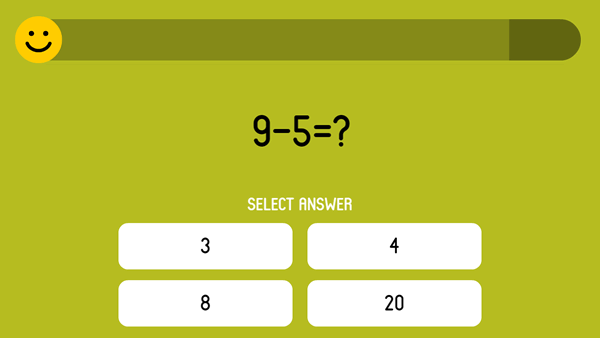# Last Math Games

## Math Mahjong Relax

Welcome to online Math Mahjong Relax. Math Mahjong Relax is a traditional solitaire game where you have to take apart the construction made of dice. The game helps to improve your attention, speed and math skills. Dice differ...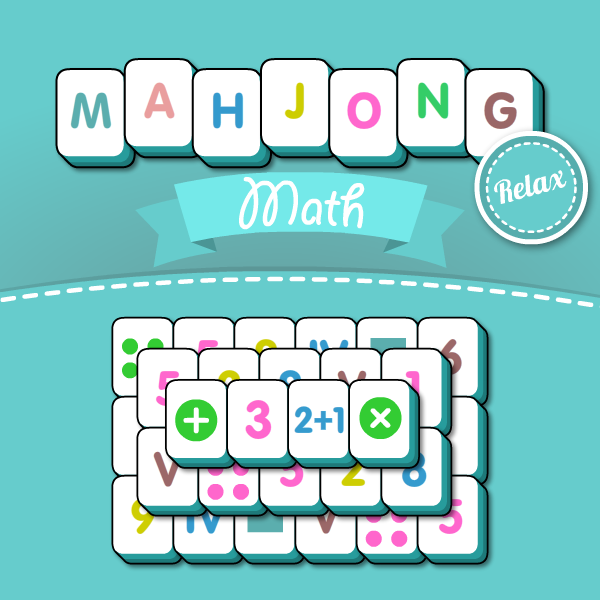## Math Number Challenge

In this game, you need be finding figures for speed. It looks quite easy for the first time. However, at every level new tasks appear complicating the search for numbers. In the game, you will learn figures in the decimal system. You will find out how ...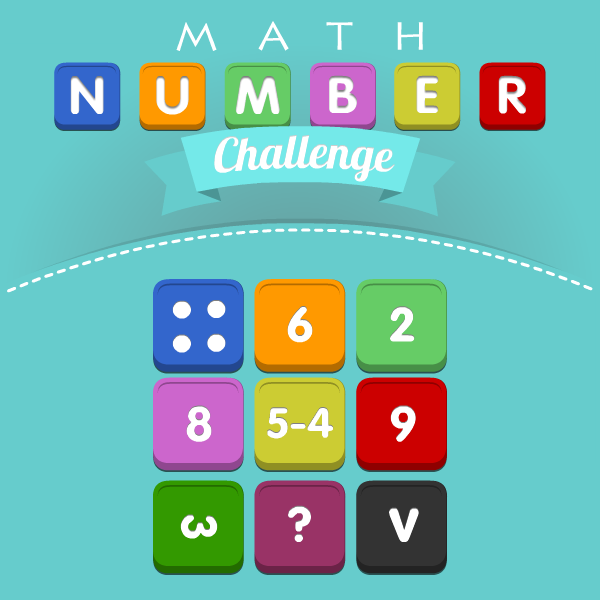## Math Pixel Puzzle

Math pixel puzzle is a very unusual game. The rules are very simple. You will see an image with pixels of various colors. After three seconds, the pixels fly apart in 3D space. Your task is to rotate the space so that ...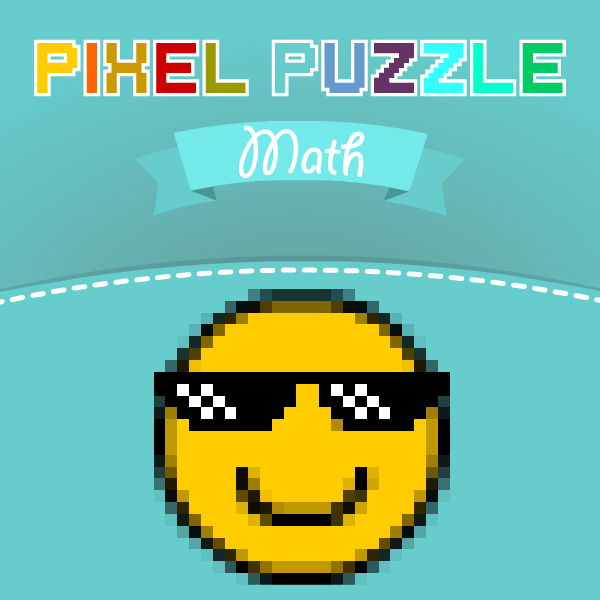# Last Math Tests

## Test to define the element in patterns with geometric figures.

It's time to try finding patterns in real time. First of all, you will see a sequence of geometric figures different in color, shape and size. Try to find the pattern for each property and to define the element hidden behind the question mark. If y...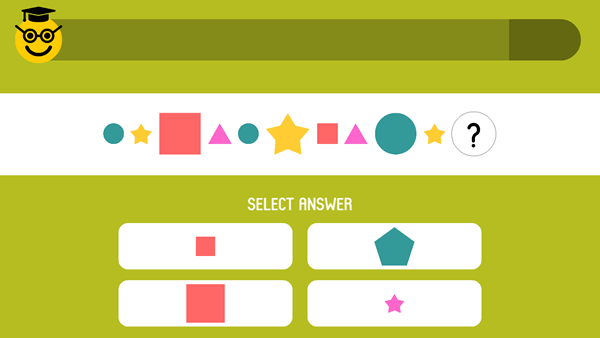## Test to define the element in patterns with sweets.

In this test, you will need to find sweets behind a question mark. All the sweets vary in color and size. The main feature of the test is that sweets can be hidden anywhere within the sequence. You should be very attentive. The task is pretty complicat...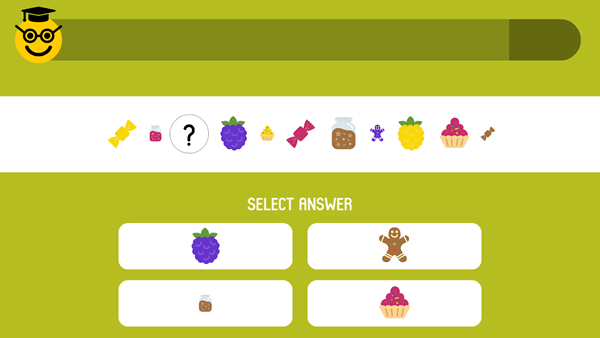## Test on the finger-counting

In this test you need to count the number of fingers on your hands and choose the right answer. You'll get 10 questions online. You'll get a score at the end. Hurry up - the time is limited for each qu...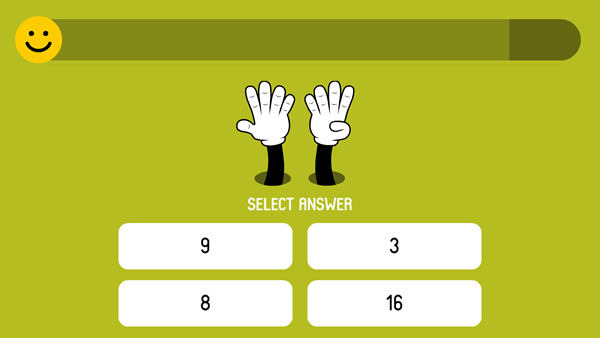## Test on the arithmetic operation division

In this test you need to solve tests on the arithmetic operation division and choose the right answer. You'll get 10 questions online. You'll get a score at the end. Hurry up - the time is limited for each ques...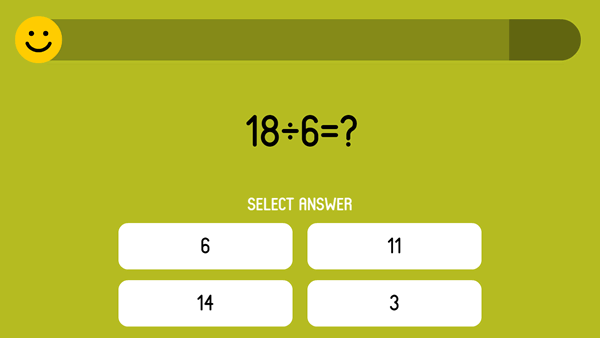## Test on the arithmetic operation multiplication

In this test you need to solve tests on the arithmetic operation multiplication and choose the right answer. You'll get 10 questions online. You'll get a score at the end. Hurry up - the time is limited for eac...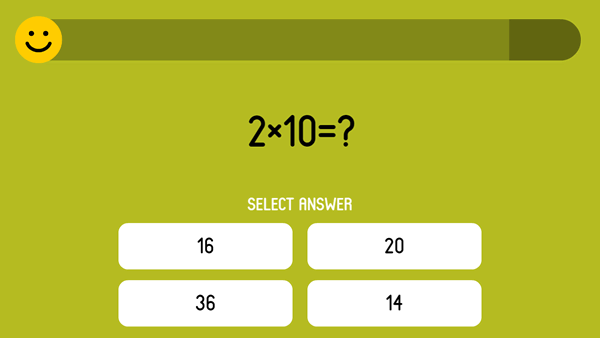## Test on the arithmetic operation minus

In this test you need to solve tests on the arithmetic operation minus and choose the right answer. You'll get 10 questions online. You'll get a score at the end. Hurry up - the time is limited for each questio...